# Resources tagged with: Similarity and congruence

Filter by: Content type:
Age range:
Challenge level:

### There are 51 results

Broad Topics > Angles, Polygons, and Geometrical Proof > Similarity and congruence### Golden Triangle

##### Age 16 to 18Challenge Level

Three triangles ABC, CBD and ABD (where D is a point on AC) are all isosceles. Find all the angles. Prove that the ratio of AB to BC is equal to the golden ratio.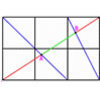##### Age 16 to 18Challenge Level

A new problem posed by Lyndon Baker who has devised many NRICH problems over the years.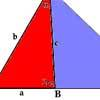### Double Angle Triples

##### Age 16 to 18Challenge Level

Try out this geometry problem involving trigonometry and number theory### Pent

##### Age 14 to 18Challenge Level

The diagram shows a regular pentagon with sides of unit length. Find all the angles in the diagram. Prove that the quadrilateral shown in red is a rhombus.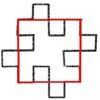### Squareflake

##### Age 16 to 18Challenge Level

A finite area inside and infinite skin! You can paint the interior of this fractal with a small tin of paint but you could never get enough paint to paint the edge.### Pentakite

##### Age 14 to 18Challenge Level

ABCDE is a regular pentagon of side length one unit. BC produced meets ED produced at F. Show that triangle CDF is congruent to triangle EDB. Find the length of BE.### Pentabuild

##### Age 16 to 18Challenge Level

Explain how to construct a regular pentagon accurately using a straight edge and compass.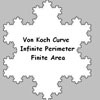### Von Koch Curve

##### Age 16 to 18Challenge Level

Make a poster using equilateral triangles with sides 27, 9, 3 and 1 units assembled as stage 3 of the Von Koch fractal. Investigate areas & lengths when you repeat a process infinitely often.##### Age 14 to 16Challenge Level

Two ladders are propped up against facing walls. The end of the first ladder is 10 metres above the foot of the first wall. The end of the second ladder is 5 metres above the foot of the second. . . .### Making Sixty

##### Age 14 to 16Challenge Level

Why does this fold create an angle of sixty degrees?### Wedge on Wedge

##### Age 14 to 16Challenge Level

Two right-angled triangles are connected together as part of a structure. An object is dropped from the top of the green triangle where does it pass the base of the blue triangle?### Triangle in a Trapezium

##### Age 11 to 16Challenge Level

Can you find and prove the relationship between the area of a trapezium and the area of a triangle constructed within it?##### Age 14 to 16Challenge Level

Find the area of the shaded region created by the two overlapping triangles in terms of a and b?### Chord

##### Age 16 to 18Challenge Level

Equal touching circles have centres on a line. From a point of this line on a circle, a tangent is drawn to the farthest circle. Find the lengths of chords where the line cuts the other circles.### Another Triangle in a Triangle

##### Age 16 to 18Challenge Level

Can you work out the fraction of the original triangle that is covered by the green triangle?### A Sameness Surely

##### Age 14 to 16Challenge Level

Triangle ABC has a right angle at C. ACRS and CBPQ are squares. ST and PU are perpendicular to AB produced. Show that ST + PU = AB### Figure of Eight

##### Age 14 to 16Challenge Level

On a nine-point pegboard a band is stretched over 4 pegs in a "figure of 8" arrangement. How many different "figure of 8" arrangements can be made ?### Napkin

##### Age 14 to 16Challenge Level

A napkin is folded so that a corner coincides with the midpoint of an opposite edge . Investigate the three triangles formed .### Triangle Midpoints

##### Age 14 to 16Challenge Level

You are only given the three midpoints of the sides of a triangle. How can you construct the original triangle?### Partly Circles

##### Age 14 to 16Challenge Level

What is the same and what is different about these circle questions? What connections can you make?### Nicely Similar

##### Age 14 to 16Challenge Level

If the hypotenuse (base) length is 100cm and if an extra line splits the base into 36cm and 64cm parts, what were the side lengths for the original right-angled triangle?### Pythagoras Proofs

##### Age 14 to 16Challenge Level

Can you make sense of these three proofs of Pythagoras' Theorem?### Triangular Slope

##### Age 14 to 16 ShortChallenge Level

Can you find the gradients of the lines that form a triangle?### Fit for Photocopying

##### Age 14 to 16Challenge Level

Explore the relationships between different paper sizes.### Sitting Pretty

##### Age 14 to 16Challenge Level

A circle of radius r touches two sides of a right angled triangle, sides x and y, and has its centre on the hypotenuse. Can you prove the formula linking x, y and r?### Squirty

##### Age 14 to 16Challenge Level

Using a ruler, pencil and compasses only, it is possible to construct a square inside any triangle so that all four vertices touch the sides of the triangle.### Triangle in a Triangle

##### Age 14 to 16Challenge Level

Can you work out the fraction of the original triangle that is covered by the inner triangle?### Kite in a Square

##### Age 14 to 16Challenge Level

Can you make sense of the three methods to work out the area of the kite in the square?### Arrh!

##### Age 14 to 16Challenge Level

Triangle ABC is equilateral. D, the midpoint of BC, is the centre of the semi-circle whose radius is R which touches AB and AC, as well as a smaller circle with radius r which also touches AB and AC. . . .### Points in Pairs

##### Age 14 to 16Challenge Level

Move the point P to see how P' moves. Then use your insights to calculate a missing length.### From All Corners

##### Age 14 to 16Challenge Level

Straight lines are drawn from each corner of a square to the mid points of the opposite sides. Express the area of the octagon that is formed at the centre as a fraction of the area of the square.### Slippage

##### Age 14 to 16Challenge Level

A ladder 3m long rests against a wall with one end a short distance from its base. Between the wall and the base of a ladder is a garden storage box 1m tall and 1m high. What is the maximum distance. . . .### Look Before You Leap

##### Age 14 to 16Challenge Level

Can you spot a cunning way to work out the missing length?### Trapezium Four

##### Age 14 to 16Challenge Level

The diagonals of a trapezium divide it into four parts. Can you create a trapezium where three of those parts are equal in area?### Doubly Isosceles

##### Age 14 to 16 ShortChallenge Level

Find the missing distance in this diagram with two isosceles triangles### Matter of Scale

##### Age 14 to 16Challenge Level

Prove Pythagoras' Theorem using enlargements and scale factors.### Sierpinski Triangle

##### Age 16 to 18Challenge Level

What is the total area of the triangles remaining in the nth stage of constructing a Sierpinski Triangle? Work out the dimension of this fractal.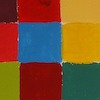### The Square Under the Hypotenuse

##### Age 14 to 16Challenge Level

Can you work out the side length of a square that just touches the hypotenuse of a right angled triangle?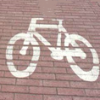### It Depends on Your Point of View!

##### Age 14 to 16Challenge Level

Anamorphic art is used to create intriguing illusions - can you work out how it is done?### Same Length

##### Age 11 to 16Challenge Level

Construct two equilateral triangles on a straight line. There are two lengths that look the same - can you prove it?### Angle Trisection

##### Age 14 to 16Challenge Level

It is impossible to trisect an angle using only ruler and compasses but it can be done using a carpenter's square.### Fitting In

##### Age 14 to 16Challenge Level

The largest square which fits into a circle is ABCD and EFGH is a square with G and H on the line CD and E and F on the circumference of the circle. Show that AB = 5EF. Similarly the largest. . . .### Parallel Universe

##### Age 14 to 16Challenge Level

An equilateral triangle is constructed on BC. A line QD is drawn, where Q is the midpoint of AC. Prove that AB // QD.### Strange Rectangle

##### Age 16 to 18Challenge Level

ABCD is a rectangle and P, Q, R and S are moveable points on the edges dividing the edges in certain ratios. Strangely PQRS is always a cyclic quadrilateral and you can find the angles.### Bus Stop

##### Age 14 to 16Challenge Level

Two buses leave at the same time from two towns Shipton and Veston on the same long road, travelling towards each other. At each mile along the road are milestones. The buses' speeds are constant. . . .### All Tied Up

##### Age 14 to 16Challenge Level

A ribbon runs around a box so that it makes a complete loop with two parallel pieces of ribbon on the top. How long will the ribbon be?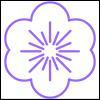### Flower

##### Age 16 to 18Challenge Level

Six circles around a central circle make a flower. Watch the flower as you change the radii in this circle packing. Prove that with the given ratios of the radii the petals touch and fit perfectly.### Two Triangles in a Square

##### Age 14 to 16Challenge Level

Given that ABCD is a square, M is the mid point of AD and CP is perpendicular to MB with P on MB, prove DP = DC.### Folding Squares

##### Age 14 to 16Challenge Level

The diagonal of a square intersects the line joining one of the unused corners to the midpoint of the opposite side. What do you notice about the line segments produced?### Folding Fractions

##### Age 14 to 16Challenge Level

What fractions can you divide the diagonal of a square into by simple folding?